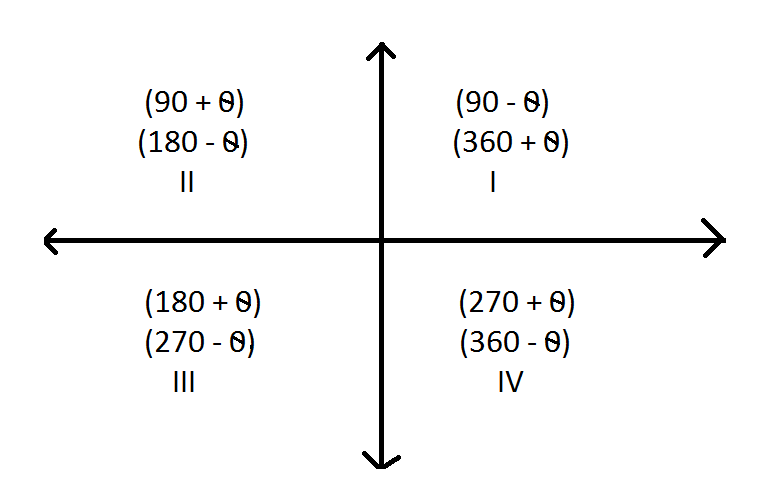QuestionAnswers

# Find the value of trigonometric functions.Verified
128.7k+ views
Hint: Such question is to be done on the basic quadrant rule of trigonometry.
In the first quadrant the values of $\sin ,\cos ,\tan$and $\cot$ are positive. In the second quadrant $\sin \,and\,\cos ec$ are positive. In the third quadrant $\tan$ and the$\cot$and positive and in the fourth quadrant $\cos$ and $\sec$ are positive.
Trigonometric ratio’s do change at odd multiples of ${90^0},{270^0},{450^0}$etc
Example $\operatorname{Sin} (90 - \theta ) = \operatorname{Cos} \theta$
At odd multiples of ${90^0}\operatorname{Sin}$ change to $\cos$ and $\cos$ to $\sin ,\tan$ changes to $\cot$ and $\cot$changes to $\tan ,$ $\cos es$ changes to $\sec$ and $\sec$ changes to $\cos es$
Trigonometric ratios do not change at even multiples of ${90^0}\,$ i.e. $180,360$etc.
They remain same
In first quadrant$\theta$ lies between $O < \theta < 90$
In second quadrant$\theta$ lies between $90 < O < 180$
In third quadrant$\theta$ lies between $180 < \theta < 270$
In fourth quadrant$\theta$ lies between $360 < \theta < 270$Therefore,

$\sin {765^0}.........(1)$
We can write $765 = 720 + 45$
$\Rightarrow 765 = 2 \times 360 + 45...........eqn(2)$
We have, $(\sin ){765^0} = \operatorname{Sin} \left[ { = 2(360) + 45} \right]$
We know $360 + \theta$ lies in the first quadrant and in the first quadrant all trigonometric ratios are positive.
Also $360$ is an even multiple of $90$
Hence $\operatorname{Sin} (360 + \theta ) = \operatorname{Sin} \theta$
i.e. $Sin\left[ {2(360) + 45)} \right] = \operatorname{Sin} 45$
Note: The quadrant rule can be used to find the trigonometric ratio of any angle. In the first round it covers ${360^0}$. Second ${720^0}$ and so on.